# Community

Blog Events Webinars Tutorials Forum
×
Community Blog PyCon China 2018: In-Depth Analysis of Mars

# PyCon China 2018: In-Depth Analysis of Mars

This article shares the "What, Why, and How" of Mars, presented at the PyCon China 2018 conference in Beijing, Chengdu, and Hangzhou.

We shared our latest project, Mars, a matrix-based unified computing framework, at the main venue of the PyCon China 2018 conference in Beijing, as well as at its sub-venues in Chengdu and Hangzhou. This article explains the shared content at the PyCon China 2018 conference in the form of text.

For those who have never heard of Mars, you may wonder what Mars is, what Mars can do, and how Mars work. Today, we answer these questions from the experience with Mars and by using an example.

## Background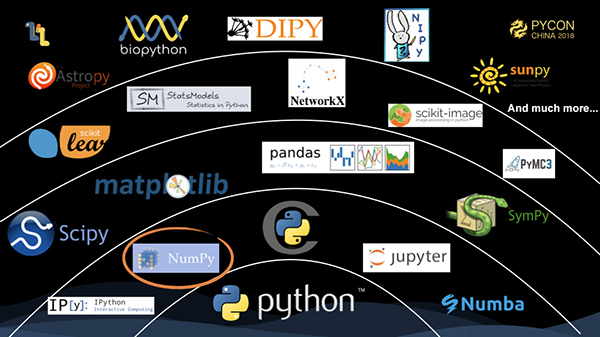First, this is a panorama of the scipy technology stack. NumPy is the foundation. It provides the data structure of multi-dimensional arrays and various computations that operate upon it. Further up, attentions should be paid to the following: scipy, which is mainly for various scientific computing operations; and pandas, with DataFrame as the core concept. It provides functions, such as processing and cleaning, for table-type data. On the next level, the classical library, scikit-learn, is one of the most well-known machine learning frameworks. At the top level is a variety of libraries for vertical fields, such as astropy, which is mainly oriented to the astronomical field, and biopython, which is oriented to the biological field.

From the scipy technology stack, you can see that numpy plays a key role, and a large number of upper-level libraries use the data structure and computation provided by numpy.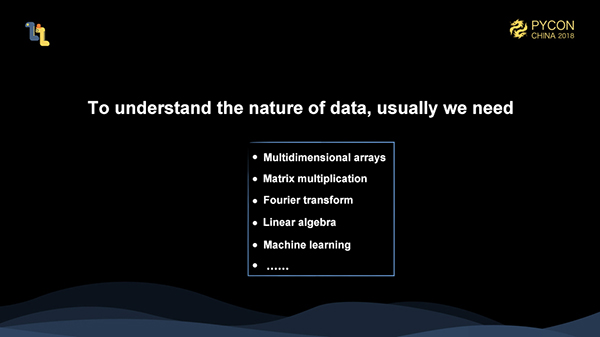Real-world data is not as simple as the two-dimensional data, such as tables. Most of the time, we often have to deal with multi-dimensional data. For example, for common image processing, the data to be processed includes the number of pictures, the length and width of the pictures, and RGBA channels. This comprises the four-dimensional data. Such examples are too numerous to enumerate. With such multi-dimensional processing capabilities, we have the ability to deal with a variety of more complex or even scientific fields. Meanwhile, multi-dimensional data itself contains two-dimensional data, so we also have the ability to process table-type data.

In addition, if we need to explore the inner nature of the data, it is absolutely not sufficient to just perform statistics and other similar operations on the table data. We need to use deeper "mathematical" methods, such as matrix multiplication and Fourier transformations, to analyze the data at a deeper level. As numpy is a library for numerical computation, we think it, together with various upper-level libraries, is very suitable for meeting these needs.

## Why Mars?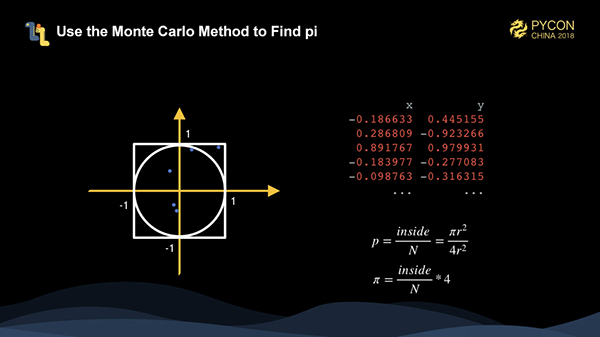So, why do we need to work on the Mars project? Let's take an example.

We try to use the Monte Carlo method to calculate pi. The method is actually very simple, which is to solve a particular problem using random numbers. As shown in the figure, we have a circle with a radius of 1 and a square with a side length of 2. We generate many random points, and then we can calculate the value of pi using the formula in the lower right corner, that is, 4 multiplied by the number of points falling in the circle divided by the total number of points. The more randomly generated points, the more accurate the calculated pi is.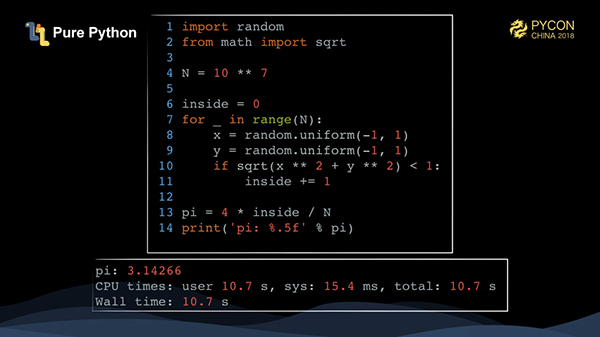This is very easily implemented using pure Python. We only need to traverse N times, generate X and Y points and calculate whether it falls within a circle. It takes more than 10 seconds to run 10 million points.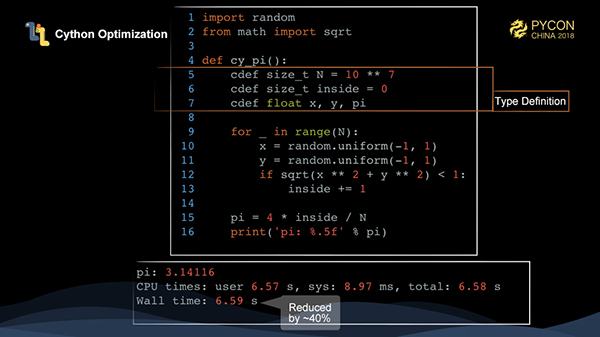Cython is a common way to reduce the time required to execute the Python code. Cython defines a superset of the Python language, translates the language into c/c++, and then compiles it to speed up the execution. Here, we have added several types of variables, and you can see a 40% performance improvement over pure Python.

Cython has now become a standard configuration for Python projects, and the core third-party library of Python basically uses Cython to speed up Python code execution.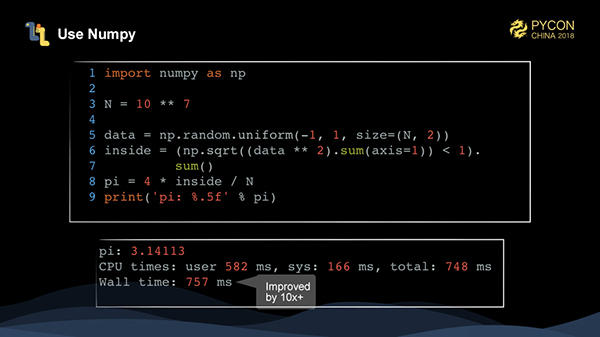The data in this example is of one type, so we can use a specialized numerical computation library to speed up the execution of this task very quickly through vectorization. Numpy is the inevitable choice. With numpy, what we need is an array-oriented mindset that reduces loops. We first use numpy.random.uniform to generate a two-dimensional array of N 2, then data * 2 to square all the data in the array, and then sum(axis=1) to sum axis=1 (that is, in the row direction).

At this time, we obtain a vector of the length N, then we use numpy.sqrt to find the square of each value of the vector. If the result < 1, we obtain a boolean vector to determine whether each point falls into the circle or not. We can find out the total number of points by following a sum at the end. It may be difficult to get started with numpy at first, but after becoming more familiar with it, you should realize how convenient this method of writing can be. It is actually very intuitive.

As you can see, by using numpy, we have written simpler code, and the performance has been greatly improved, which is more than 10 times better than that of the pure Python.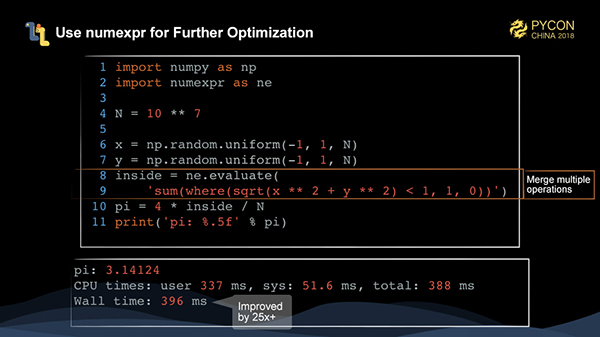Can the numpy code be optimized? The answer is yes. We use a library called numexpr to fuse several numpy operations into one operation to speed up the numpy execution.

As you can see, the performance of code optimized by numexpr is more than 25 times better than that of the pure Python code.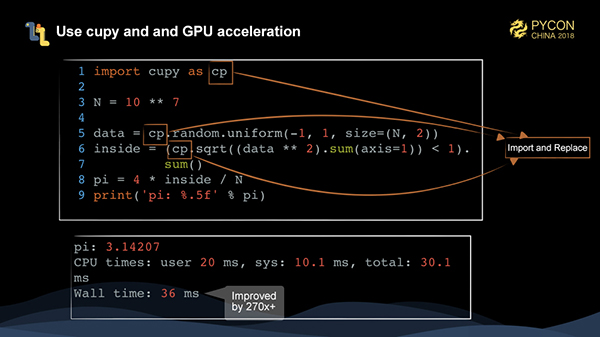At this time, the code is already running quite fast. If we have a GPU, we can use hardware to accelerate the task execution.

A library called cupy is strongly recommended for this type of task, which provides an API that is consistent with numpy. By simply replacing the import, numpy code can be run on NVIDIA graphics card.

At this time, the performance has been greatly improved by more than 270 times. It is quite remarkable.

To improve the accuracy of the Monte Carlo method, we have increased the amount of computation by a factor of 1,000. What happens in this situation?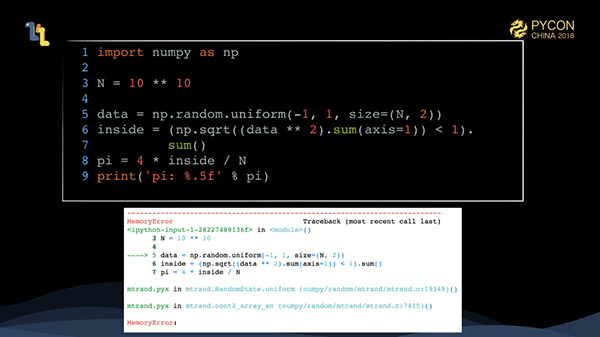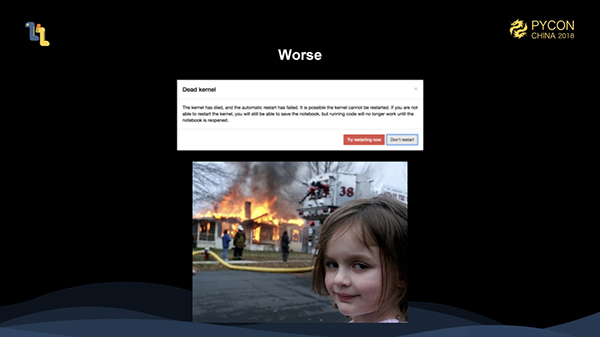Yes, this is the memory overflow (OutOfMemory) we encounter from time to time. Worse still, in jupyter, OutOfMemory sometimes causes processes to be killed and even results in previous runs being lost.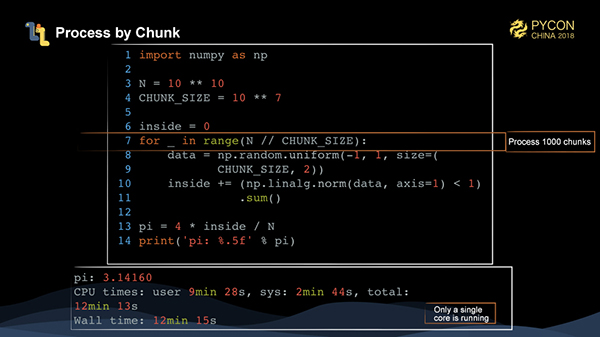The Monte Carlo method is relatively easy to handle. We simply divide the problem into 1,000 blocks, each solving 10 million entries of data, and then write a loop and make a sum. However, the whole computing time is over 12 minutes, which is too slow.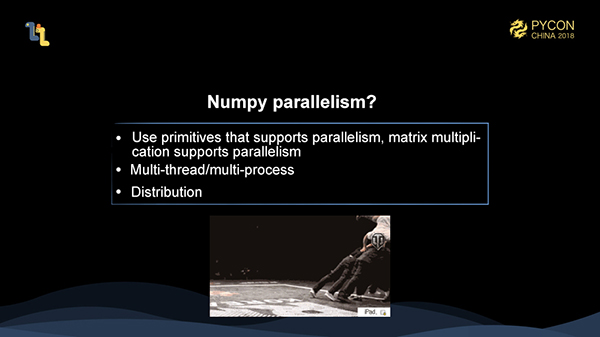At this point, we can find that, during the entire operation, only one CPU is actually working, while other cores are not. So, how do we parallelize operations within numpy?

Some operations in numpy can be parallelized, such as using tensordot for matrix multiplication. Most other operations cannot use multiple cores. To parallelize operations within numpy, we can:

2. Use a distributed method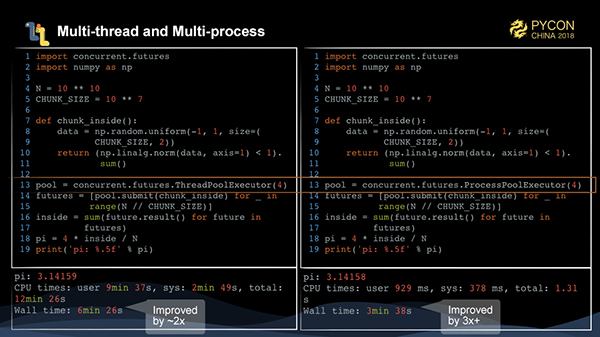It is still very easy to rewrite the task of calculating pi by the Monte Carlo method into a implementation of multi-threads and multi-processes. We write a function to process 10 million data entries. Then, we submit this function 1,000 times through ThreadPoolExecutor and ProcessPoolExecutor of concurrent.futures respectively to be executed by multi-threads and multi-processes. We can see that the performance can be improved by 2 or 3 times.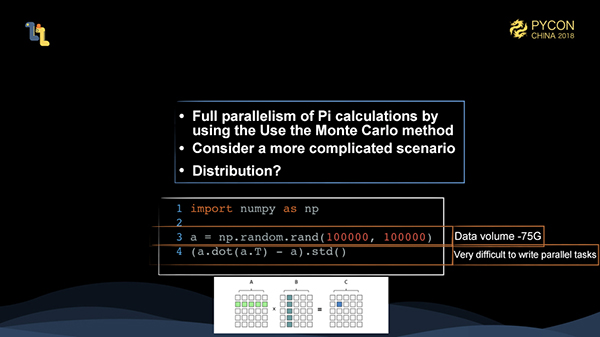However, calculating pi using the Monte Carlo method is very easily written in parallel, so we need to consider more complicated situations.

``````import numpy as np

a = np.random.rand(100000, 100000)
(a.dot(a.T) - a).std()``````

We have created a matrix "a" of 100,000 * 100,000, and the input is about 75 GB. We multiply matrix "a" by the transposition of "a", subtract "a" itself, and finally calculate the standard deviation. The input data of this task can't easily be stuffed into memory, and the subsequent parallel writing operation is even more difficult.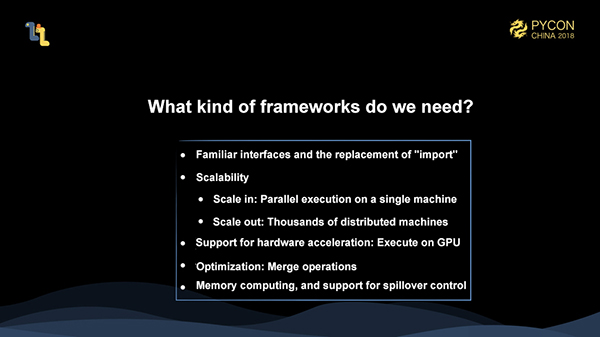This brings us to the question - what kind of framework do we need? Ideally, our framework should be able to satisfy the following requirements:

• It should provide familiar interfaces. Cupy, for example, can parallelize the code originally written in numpy by simply replacing the "import".
• It should be scalable. Even if it is as small as a stand-alone machine, it can use multi-core parallelism. If it is as large as a large cluster, it can support the scale of thousands of machines to handle tasks together in a distributed manner.
• It should support the use of hardware, such as GPUs, to accelerate task execution.
• It should support various optimizations, such as fusion, and can use some libraries to accelerate the operation of fusion.
• Although we only perform in-memory computing, we do not want to run out of memory on a single machine or cluster, otherwise the task fails. We should dump temporarily unused data into storage, such as onto disks, to ensure that the entire computation can be completed even if insufficient memory is available.

## What Is Mars and What Is It Capable of Doing?

Mars is a framework that intends to solve these type of problems. Currently, Mars includes tensors: the distributed multi-dimensional matrix computing.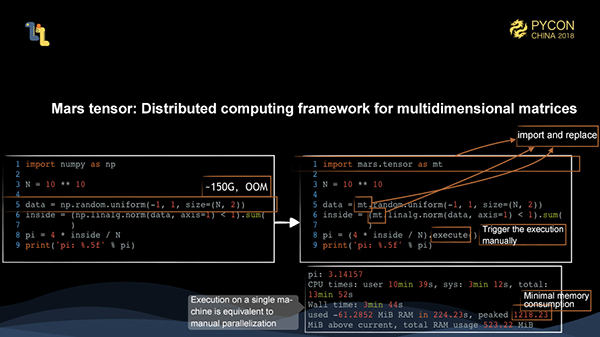The task scale for calculating pi using the Monte Carlo method with the size of 10 billion is 150 GB, which causes OOM. By using the Mars tensor API, you only need to replace `import numpy as np` with `import mars.tensor as mt`, and the subsequent computations are exactly the same. However, there is one difference. A Mars tensor needs to be triggered by `execute`, which has the advantage of optimizing the entire intermediate process as much as possible, such as fusion. This method is not very helpful for debugging, but we will provide the eager mode in the future which can trigger the computation for each step, which is exactly the same as with numpy code.

As shown above, this computation time is equivalent to that of parallel writing, and the peak memory usage is just a little bit more than 1 GB. This shows that we can achieve full parallelism and save the memory usage through Mars tensors.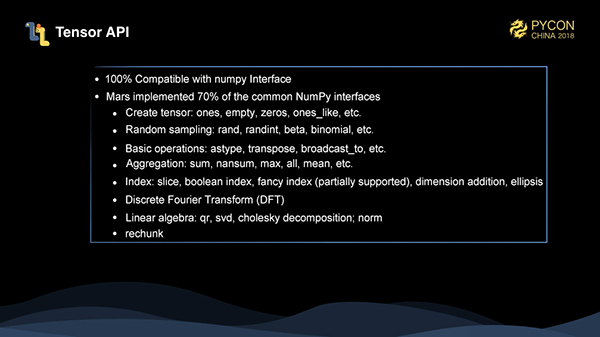Currently, Mars has implemented 70% of the common numpy interfaces. For a complete list, see here. We have been working hard to provide more numpy and scipy interfaces. We have recently completed our support for Inverse Matrix computing.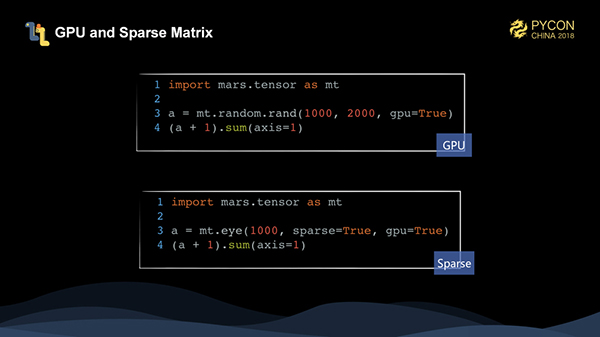Mars tensors also support GPUs and sparse matrices. eye is used to create a unit diagonal matrix, which only has a value of 1 on the diagonal and wastes storage if stored densely. Currently, Mars tensors only support two-dimensional sparse matrices.

## How Does Mars Achieve Parallelization and Save on Memory Usage?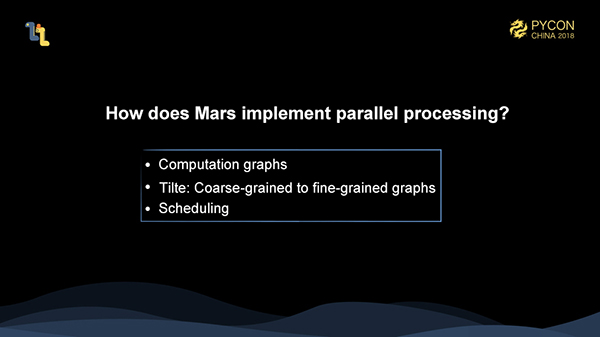Like all the dataflow frameworks, Mars also has the concept of Computational Graphs. The difference is that Mars contains the concept of coarse-grained and fine-grained graphs. The client-written code generates a coarse-grained graph on the client. After it is submitted to the server, there is a tiling process and the coarse-grained graph is tiled into a fine-grained graph. Then we schedule the fine-grained graph to execute.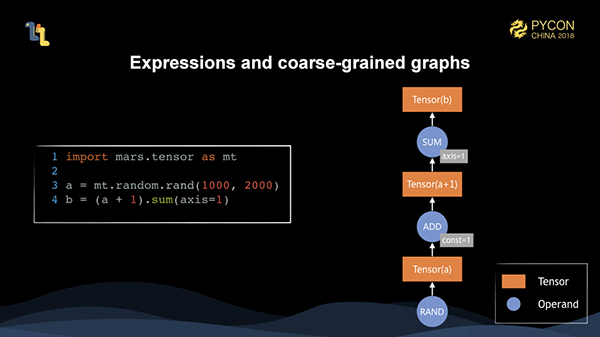Here, the client-written code is expressed in memory as a coarse-grained graph composed of tensors and operands.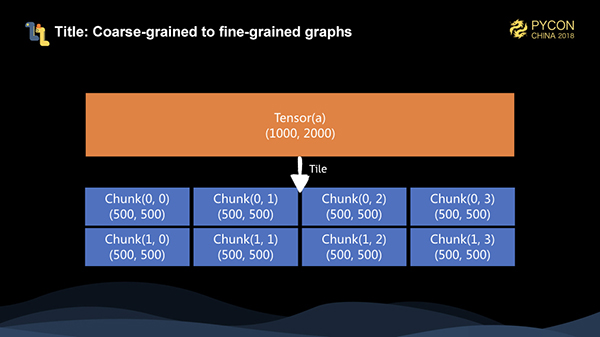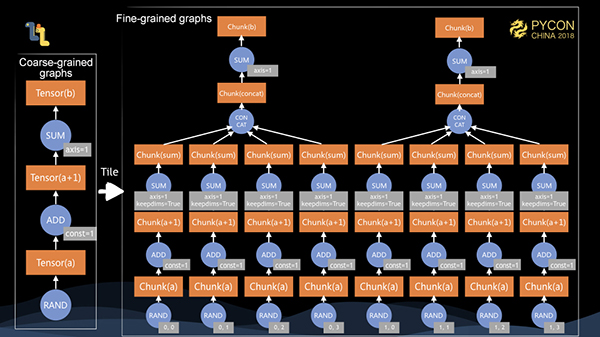When the client calls the `execute` method, the coarse-grained graph is serialized to the server. After deserialization, we tile the graph into a fine-grained graph. For a matrix of 10002000, assuming that the chunk size on each dimension is 500, then it is tiled into 24 for a total of 8 chunks.

Later, we perform tiling operations for each implemented operands, namely operators, tiling a coarse-grained graph into a fine-grained graph. At this time, we can see that if there are 8 cores in a stand-alone machine, then we can execute the entire fine-grained graph in parallel. In addition, given 12.5% of the memory size, we can complete the computation of the entire graph.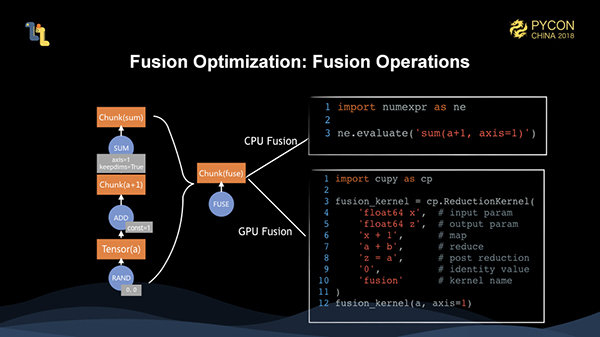However, before we actually start the execution, we fuse the entire graph, which means that the fusion is optimized. When the three operations are actually executed, they are fused into one operator. For different execution targets, we use the fusing support of numexpr and cupy to fuse and execute CPU and GPU operations respectively.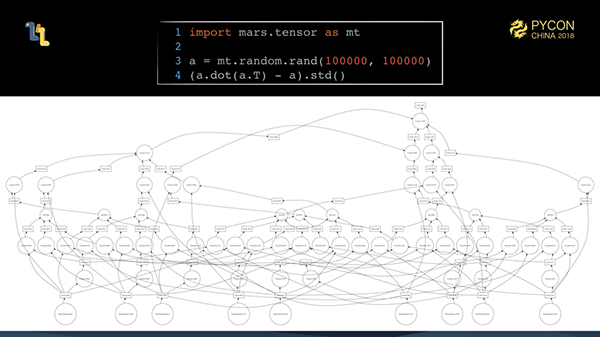The examples above are all tasks that can be easily executed in parallel. As we mentioned earlier, the fine-grained graph generated after tiling is actually very complex. In real-world computing scenarios, such tasks are actually a lot.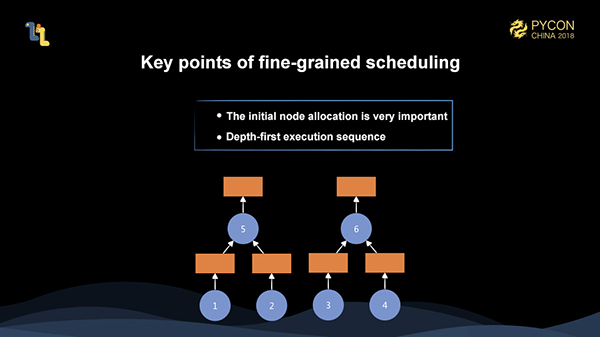To fully schedule the execution of these complex fine-grained graphs, we must meet some basic principles to make the execution efficient enough.

First, the allocation of initial nodes is very important. For example, in the figure above, suppose we have two workers. If we allocate 1 and 3 to one worker, and 2 and 4 to another worker, then when 5 or 6 are scheduled, they need to trigger a remote data pull, resulting in greatly reduced execution efficiency. If we initially allocate 1 and 2 to one worker, and 3 and 4 to another worker, the execution is very efficient. The allocation of initial nodes has significant impact on the overall execution. Therefore, we need to have a global grasp of the whole fine-grained graph before we can achieve a better initial node allocation.

In addition, the policy of depth-first execution is also very important. Suppose we only have one worker at this time, and after executing 1 and 2, if we schedule 3, the memory allocated to 1 and 2 can not be released, because 5 has not been triggered yet. However, if we schedule 5 after executing 1 and 2, then the memory allocated to 1 and 2 can be released after the execution of 5, which saves the most memory during the entire execution.

Therefore, initial node allocation and depth-first execution are the two most basic principles. However, these two points alone are far from enough, because there are many challenging tasks in the overall execution scheduling for Mars. Also, this is an object we need to optimize for the long term.

## Mars Distributed Framework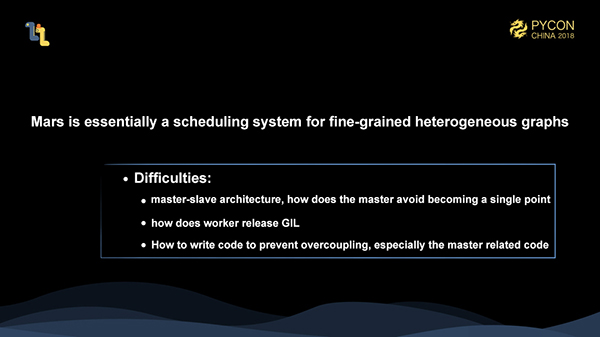As mentioned above, Mars is essentially a scheduling system for fine-grained heterogeneous graphs. We schedule fine-grained operators to various machines. In actual execution, we call libraries, such as numpy, cupy, and numexpr. We have made full use of a mature and highly optimized stand-alone library, instead of reinventing the wheel in these fields.

During this process, we may encounter some difficulties:

• We use the master-slave architecture, so how can we avoid the master becoming a single point?
• How does workers avoid GIL (Global Interpreter Lock) restrictions of Python?
• The control logic of the master is very complicated. We can easily write highly coupled and long code. But how can we decouple the code?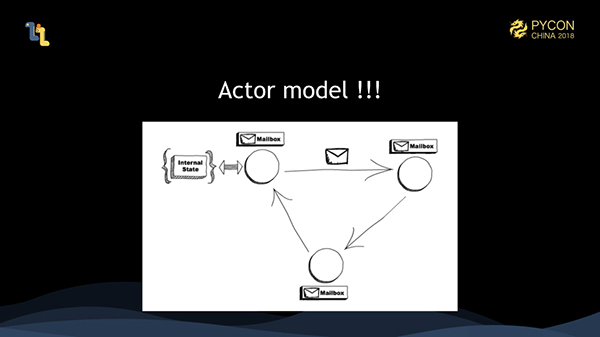Our solution is to use the actor model. The actor model defines a parallel mode. That is, everything is an actor. Each actor maintains an internal state, and they all hold a mailbox. Actors communicate with each other through messages. Messages received are placed in the mailbox. Actors retrieve messages from the mailboxes for processing, and one actor can only process one message at a time. An actor is the smallest parallel unit. An actor can only process one message at a time, so you do not need to worry about concurrency at all. Concurrency should be handled by the actor framework. Also, whether all actors are on the same machine or not becomes irrelevant in the actor model. The actor model naturally supports distributed systems provided that actors can complete message transmissions on different machines.

An actor is the smallest parallel unit. Therefore, when writing code, we can decompose the entire system into many actors, and each actor takes a single responsibility, which is somewhat similar to the idea of object-oriented programming, so that our code can be decoupled.

In addition, after the master is decoupled into actors, we can distribute these actors on different machines, so that the master is no longer a single point. At the same time, we have these actors allocated based on the consistent hash. If any scheduler crashes in the future, the actors can be reallocated and recreated based on the consistent hash to achieve the purpose of fault tolerance.

Finally, actors are run on multiple processes, and each process has many coroutines. This way, workers are not restricted by GIL.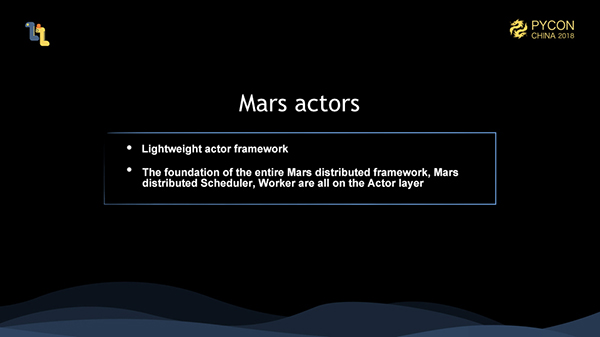JVM languages, such as Scala and Java, can use the actor framework, akka. For Python, there is no standard practice. We think that a lightweight actor framework should be able to meet our needs, and we don't need some of the advanced functions provided by akka. Therefore, we have developed Mars actors, a lightweight actor framework. The entire distributed schedulers and workers of Mars are on the Mars actors layer.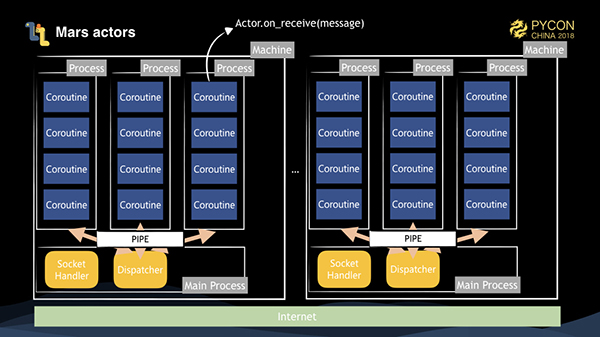This is the architecture diagram of our Mars actors. When we start the actor pool, sub-processes start several sub-processes on the basis of concurrency. The main process has a socket handler to accept messages delivered by remote socket connections, and a dispatcher object to distribute messages according to their destinations. All of the actors are created on sub-processes. When an actor receives a message for processing, we call the `Actor.on_receive(message)` method through a coroutine.

For one actor to send a message to another actor, there are three situations to consider.

1. If they are in the same process, then they can be called directly through a coroutine.
2. If they are in different processes on a machine, then the message is serialized and sent to the dispatcher of the main process through the pipeline. The dispatcher obtains the process ID of the target by unlocking the binary header information and sends it to the corresponding sub-process through the corresponding pipeline. The sub-process just triggers the message of the corresponding actor through the coroutine for processing.
3. If they are on different machines, the current sub-process sends serialized messages to the master process of the corresponding machine through the socket, and then the machine sends the messages to the corresponding sub-process through the dispatcher.

Coroutines are used as a parallel method in sub-processes, and the coroutines themselves have strong performance in IO processing, so the actor framework also has good IO performance.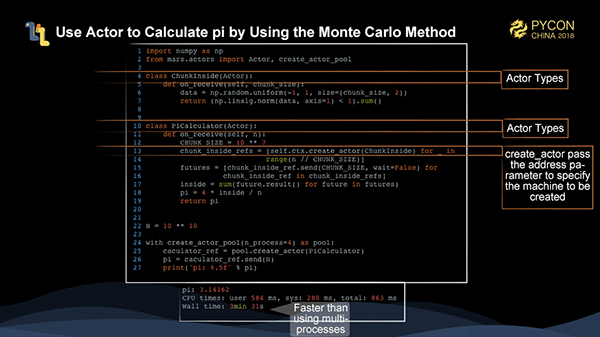The figure above shows how to calculate pi using the Monte Carlo method using only Mars actors. Two actors are defined here. One actor is the ChunkInside, which accepts the size of a chunk to calculate the number of points falling into the circle. The other actor is the PiCaculator, which accepts the total number of points to create a ChunkInside. In this example, 1,000 chunkinsides are directly created and then triggered for calculation by sending messages. Specifying address at `create_actor` allows the actors to be allocated to different machines.

As shown in the figure, the performance of just using Mars actors is faster than that of multi-processes.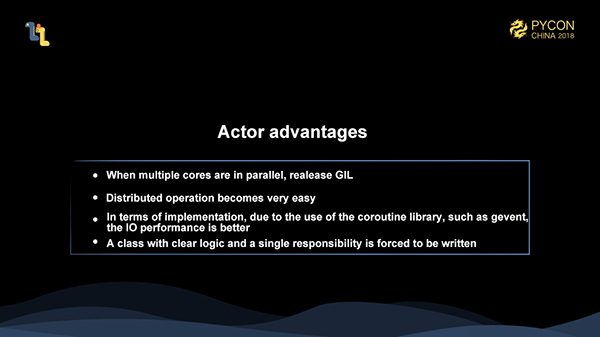To sum up, by using Mars actors, we can easily write distributed code without GIL restrictions, which improves IO efficiency. In addition, code becomes easier to maintain due to the decoupling of actors.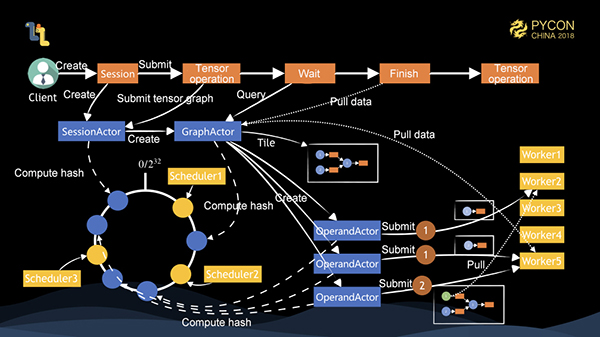Now, let's take a look at the complete distributed execution process of Mars. As shown above, we have 1 client, 3 schedulers and 5 workers. The client creates a session and the session creates a SessionActor object on the server. The object is allocated to scheduler1 through the consistent hash. At this time, the client runs a tensor. First, SessionActor creates a GraphActor, which tiles a coarse-grained graph. If there are three nodes on the graph, three OperandActors are created and allocated to different schedulers respectively. Each OperandActor controls the submission of the operand, the supervision of the task status, and the release of memory. At this time, it is found that the OperandActors of 1 and 2 do not find dependencies, and there are sufficient cluster resources, then they submit the tasks to the corresponding worker for execution. After the execution is completed, they notify 3 of the task completion. The data is executed by different workers, so the data pulling operation is triggered first and then executed after the worker to execute the tasks is determined. If the client knows that the task is completed by polling GraphActor, the operation of pulling data to local is triggered. The entire task is completed.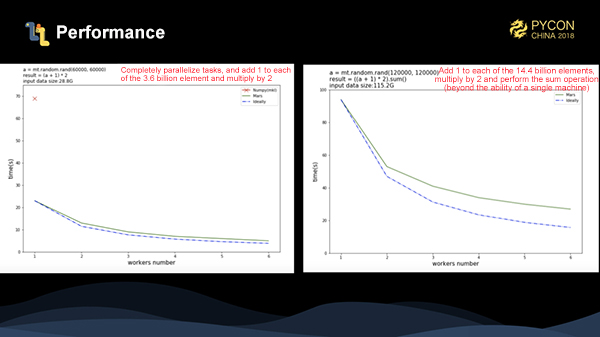We have made two benchmarks for Mars distribution. The first one is to add 1 to each element of 3.6 billion entries of data, and multiply it by 2. In the figure, the red cross is the execution time of numpy. As can be seen, the performance is several times better than that of numpy. The blue dotted line is the theoretical running time, and we can see the actual acceleration is very close to the theoretical time acceleration. In the second benchmark, we have increased the amount of data to 14.4 billion data entries. After adding 1 to each element and multiplying it by 2, we can see that the stand-alone numpy can not complete the task. At this time, we can also achieve a good acceleration ratio for this task.

## What to Expect Next?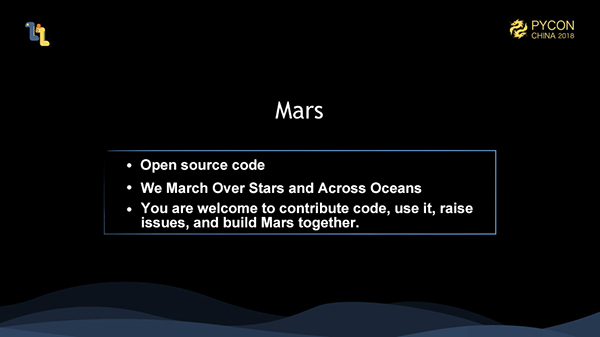The source code for Mars has already been posted on Github, allowing more prople to participate in Mars' development: https://github.com/mars-project/mars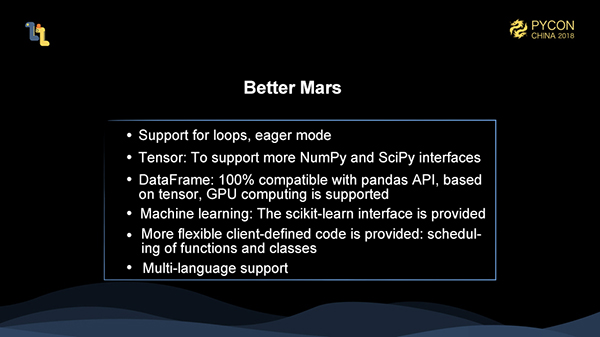As mentioned above, in the subsequent Mars development plan, we will support eager mode, allowing each step to trigger execution and improving the performance-insensitive task development and debug experience; We will support more numpy and scipy interfaces; and it is important that we provide a 100% pandas compatible interface. Based on Mars tensors, GPUs are also supported; We provide scikit-learn compatible machine learning support; We also provide the ability to schedule custom functions and classes on fine-grained graphs to enhance flexibility; Finally, our clients do not actually rely on Python and can serialize coarse-grained graphs in any language, so we can provide a multi-language client version, depending on the needs.

In short, open source is very important to us. We can't implement the parallelization of the huge scipy technology stack alone. We need everyone interested to help us build it together.

## Q&A

Finally, I would like to share some common questions and answers presented at the PyCon conferences. The general summary is as follows:

1. Mars performs some specific computations, such as SVD (Singular Value Decomposition). Here is some test data from cooperation projects with clients. The input data is a matrix of 800,000,000 * 32 for SVD. After SVD is finished, the matrices are multiplied to be compared with the original matrix. The whole computation process uses 100 workers (8 cores) and takes 7 minutes to complete.
2. When will Mars be open-sourced? A: It is already open-sourced: https://github.com/mars-project/mars
3. Will Mars be reverted to close-sourced at a later date? A: No
4. Is Mars a static graph or a dynamic graph? A: Currently, it is a static graph. After the eager mode is done, it will be able to support the dynamic graph.
5. Will Mars involve deep learning? A: Not presently0 0 0
Share on

# Related Products

• ##Quick Starts

Deploy custom Alibaba Cloud solutions for business-critical scenarios with Quick Start templates.

• ##Big Data Consulting Services for Retail Solution

Alibaba Cloud experts provide retailers with a lightweight and customized big data consulting service to help you assess your big data maturity and plan your big data journey.

• ##Big Data Consulting for Data Technology Solution

Alibaba Cloud provides big data consulting services to help enterprises leverage advanced data technology.

• ##MaxCompute

Conduct large-scale data warehousing with MaxCompute